suppressPackageStartupMessages(library(tidyverse))
library(gapminder)
knitr::opts_chunk\$set(fig.width=5, fig.height=3, fig.align="center")

# Learning Objectives

Here is what we expect you to know, corresponding to today’s lecture:

• Draw conclusions from a graph regarding the effect and confidence
• Identify the grammar components from a graph
• Identify the graph from grammar components
• Identify an appropriate graph given the variable types to be depicted
• There’s more room for flexibility when there are 3+ data variables

You are not expected to memorize the names of the plot types for your quizzes.

# Review

Last time, we mostly looked at:

1. Components: what is a statistical graphic (or “graph”)?
• Defined by the grammar of graphics
2. Tooling: how to make a plot (with ggplot2)
• Use case: appropriate plots given the types of variables to be plotted.

Elaborate:

• What are the grammar components?
• Correction from last time: aesthetic vs aesthetic mapping.
• What are the appropriate plots for…
• (First of all, what are the two main types of variables? )
• 2 numeric variables?
• 1 numeric variable?
• 1 numeric, 1 categorical variable?

We’ve also been getting set up for the other three main elements of this course

(we can think of these as a forming a flowchart: effective choice -> components + theme -> tooling -> EDA)

1. Theming: the look/decorations on a plot.
• Lecture 3 (in part)
2. Exploratory Data Analysis (EDA)
• Elaboration today (soon).
3. Plotting Effectiveness
• Chiefly Lecture 5
• Plotting principal alluded to so far: show me the data!
• Example: pinhead plots vs. violin+jitter plots.
ggplot(gapminder, aes(continent, lifeExp)) +
geom_violin() +
geom_jitter(width=0.2, alpha=0.2) +
ggtitle("Jitter + Violin Plot")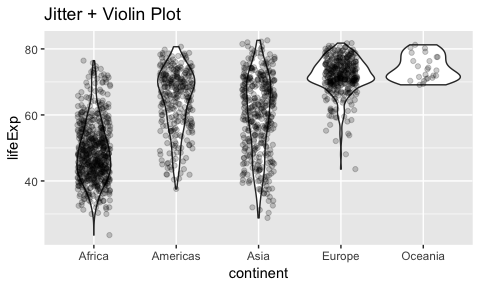gapminder %>%
group_by(continent) %>%
summarize(sd = sd(lifeExp),
mean_life_exp = mean(lifeExp)) %>%
ggplot(aes(continent)) +
geom_errorbar(aes(ymax = mean_life_exp + sd,
ymin = mean_life_exp - sd),
width=0.2) +
geom_col(aes(y=mean_life_exp)) +
ylab("lifeExp")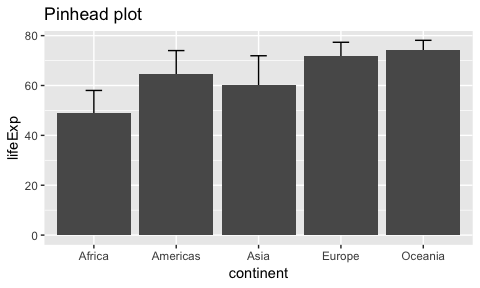# Today

• EDA
• Continue 1- and 2-variable plot types.
• 3+ variable plot types (through more aesthetics, and facetting).

We’ll fill out the lec2-worksheet.Rmd worksheet for the latter two.

# EDA

## Intro

What is exploratory data analysis (EDA)? (What is Data Science?) There’s no one definition. Generally:

• Answering questions through data exploration; as opposed to making model assumptions, model fitting, or hypothesis tests.
• Allows for us to answer questions qualitatively (not numerically). Questions are typically less specific and more “human”:
• “Has life expectancy been increasing over time?”
• vs. specifying mean
• Usually the first part of an analysis.

When reading a plot, there are generally two main components to consider:

• Effect (example: is there a relationship/trend?)
• Confidence (in the effect)

(Link to 552: estimate + uncertainty)

## Examples of EDA

Which species has the largest Sepal Width? How certain are you? (Did I need a hypothesis test for this? This is not to say that hypothesis tests are not useful)

iris %>%
ggplot(aes(Species, Sepal.Width)) +
geom_violin() +
geom_jitter(width=0.2)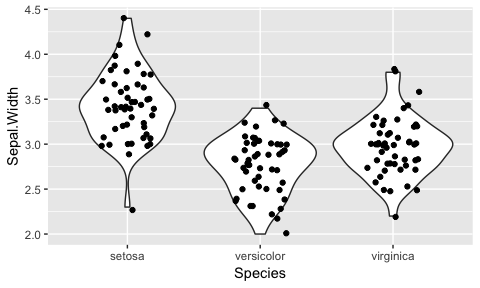Which continent will have the highest life expectancy in 2020? Will a model help you here?

gapminder %>%
group_by(continent, year) %>%
summarize(mean_life_exp = mean(lifeExp)) %>%
ggplot(aes(year, mean_life_exp)) +
geom_point() +
geom_line(aes(colour=continent, group=continent))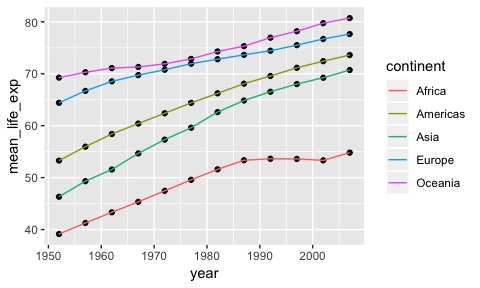Is there a relationship between GDP per capita and life expectancy? Describe the dependence. How confident are you?

ggplot(gapminder, aes(gdpPercap, lifeExp)) +
geom_point(alpha=0.2) +
scale_x_log10()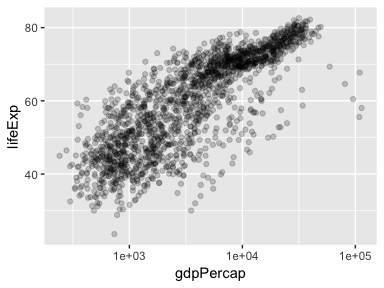How certain are you with this one?

set.seed(10)
gapminder %>%
sample_n(10) %>%
ggplot(aes(gdpPercap, lifeExp)) +
geom_point() +
scale_x_log10()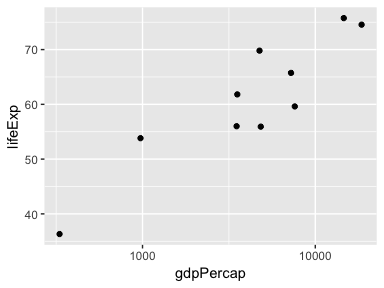Is there a relationship between the sepal and petal lengths of the setosa plant? How confident are you?

iris %>%
filter(Species == "setosa") %>%
ggplot(aes(Petal.Length, Sepal.Length)) +
geom_jitter() +
ggtitle("Species: Setosa")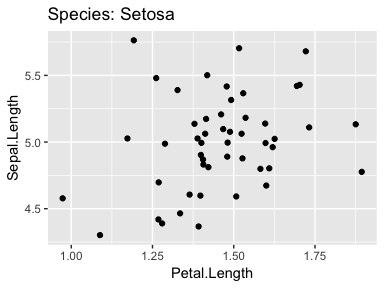Poll:

/poll Dependence strong weak none

/poll "Confidence" "highly confident", "somewhat confident", "little confidence", "no confidence"